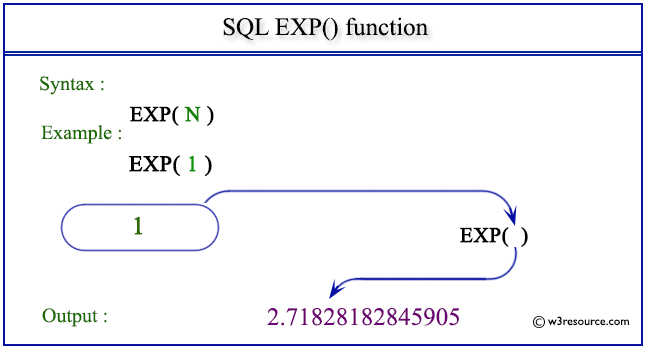# SQL EXP() function

## EXP() function

SQL EXP() function returns e raised to the n-th power(n is the numeric expression), where e is the base of a natural algorithm and the value of e is approximately 2.71828183.

Syntax:

` EXP(expression) `

Parameters:

Name Description
expression An expression which is a float or can be converted to a float.

MySQL, PostgreSQL, SQL Server, and Oracle

All of above platforms support the SQL syntax of EXP().

## SQL EXP() function: Pictorial presentationExample:

To get the e raised by 2's power from the DUAL table, the following SQL statement can be used:

``````SELECT EXP(2) AS e_to_2s_power
FROM dual;
``````

Output:

```E_TO_2S_POWER
-------------
7.3890561
```

Note: Outputs of the said SQL statement shown here is taken by using Oracle Database 10g Express Edition.

Here is a slide presentation which covers the SQL arithmetic functions.

Practice SQL Exercises